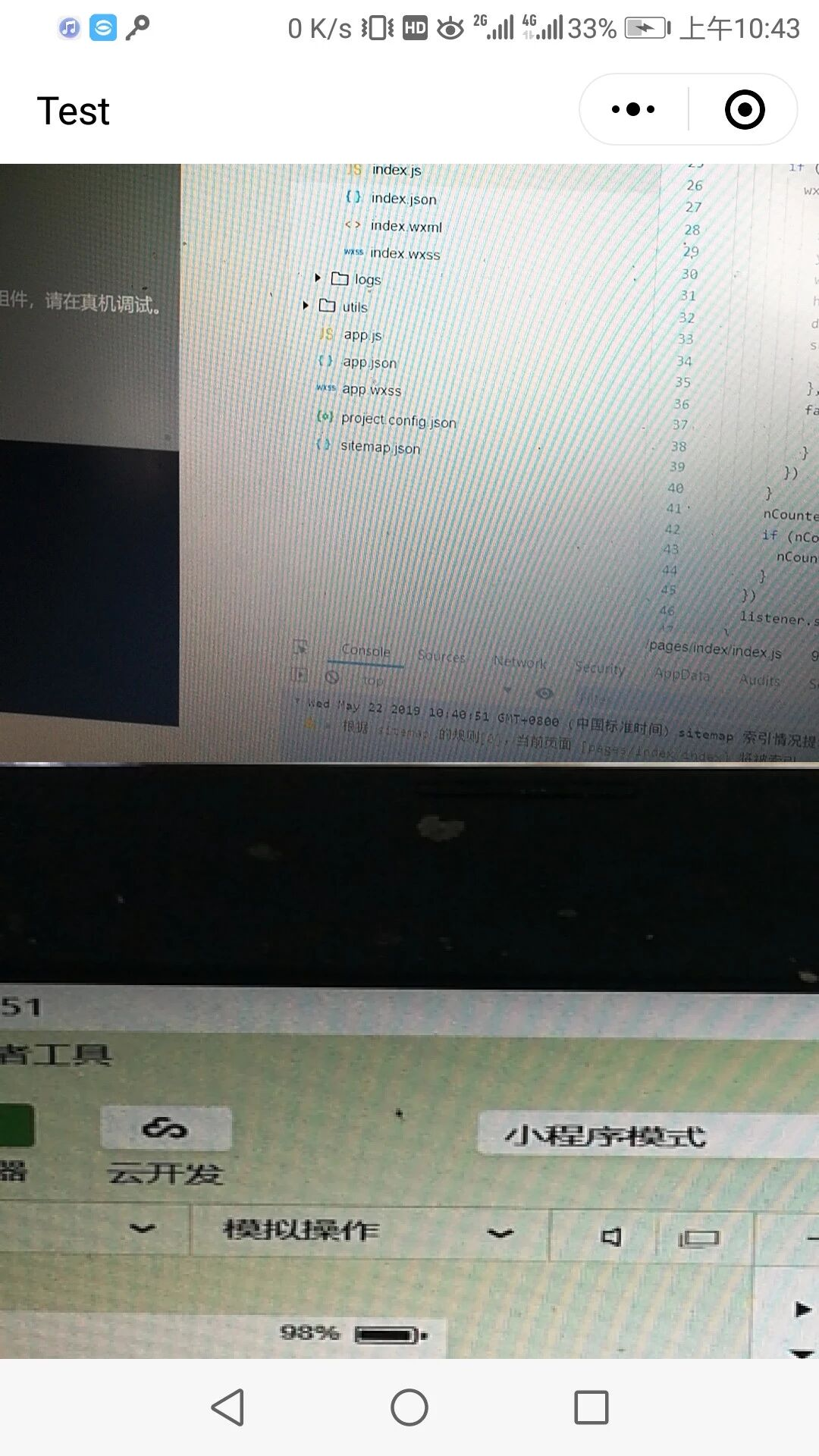# 置顶如何让onCameraFrame 获取的frame数据与相机控件显示范围一致精选热门

 `````  ````  ````  `` ``` `/**index.wxss**/` `page{``  ``width: 100%;``  ``height: 100%;``}` `.mycamera{``  ``top:0px;``  ``width: 100%;``  ``height: 50%;``  ``position: fixed;``}` `.mycanvas{``  ``top:50%;``  ``width: 100%;``  ``height: 100%;``  ``background-color: black;``  ``position: fixed;``}` `//index.js``//获取应用实例``const app = getApp()` `var` `nCounter = 0``var` `listener` `Page({``  ``data: {``  ``},` `  ``onLoad: ``function` `() {``  ``},` `  ``onReady: ``function` `(res) {``    ``var` `that = ``this``    ``var` `camera_ctx = wx.createCameraContext()  ``    ``listener = camera_ctx.onCameraFrame((frame) => {      ``      ``if` `(nCounter == 0) {``        ``wx.canvasPutImageData({``          ``canvasId: ``'mycanvas'``,``          ``x: 0,``          ``y: 0,``          ``width: frame.width,``          ``heihgt: frame.heihgt,``          ``data: ``new` `Uint8ClampedArray(frame.data),``          ``success(res) {``            ``console.log(``'OK'``)``          ``},``          ``fail(res) {``            ``console.log(``'FAIL'``)``          ``}``        ``})``      ``}``      ``nCounter++``      ``if` `(nCounter >= 10) {``        ``nCounter = 0``      ``}``    ``})``    ``listener.start()``  ``}` `})`

- 当前 Bug 的表现（可附上截图）
上面为相机控件显示的内容，下面为canvas显示onCameraFrame获取的frame数据，可见内容并不一致，主要表现是图像显示内容的范围不一致- 预期表现

- 复现路径

- 提供一个最简复现 Demo

10 个回答